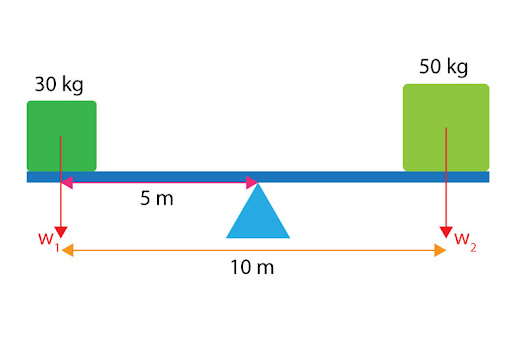# "A 10m long uniform beam is pivoted in its centre. A 30kg point mass is placed on one end of the beam. Where must a 50kg mass be placed in order to balance the beam?"For a system that is in equilibrium, we know that it has a net moment of zero - in other words:

Total clockwise moments = Total anticlockwise moments

In our given diagram, W1 offers an anticlockwise moment about the pivot, whereas W2 offers a clockwise moment.

Total Anticlockwise moment: W1 . d1 =(m1  g) . d1  = (30 . 9.81) . 5 = 1471.5 Nm

Total clockwise moment: W2  . d2  =(m2  g) . d2  =(50 . 9.81) . d2  = 490.5d2  Nm

Equating the two:

1471.5 = 490.5d

﻿d2 =1471.5/490.5=3 m